Welcome to Doom9's Forum, THE in-place to be for everyone interested in DVD conversion. Before you start posting please read the forum rules. By posting to this forum you agree to abide by the rules.Doom9's ForumHDR ColorBars - bug at transitions ?
 User Name Remember Me? Password
 Register FAQ Calendar Search Today's Posts Mark Forums ReadThread Tools Search this Thread Display Modes18th September 2021, 01:33   #21  |  Link
poisondeathray
Registered User

Join Date: Sep 2007
Posts: 4,984
Quote:
 Originally Posted by DTLCan you show example of that shader output ? I do not have vapoursynth.
This is FFV1, YUV444P16 output of placebo.Resample Sinc 16 tap. You can open in AVS with LWLibavVideoSource, or FFVideoSource. It looks worse to me. FMTC was bit identical to avs version

avs input to vpy script
Code:
```ColorBarsHD(9600,1000, pixel_type="YV24")
ConvertBits(16) # YUV 444 16
Crop(0,0,9600,500)

GaussResize(width/10,height/10, p=10)```
vpy
Code:
`clip = core.placebo.Resample(clip, width=clip.width*4, height=clip.height*4, filter ="sinc", param1=16)`

Quote:
 Also I found more execution non-stability of SincResize at different CPUs ans OS version
Maybe different path execution, SIMD, instruction sets ? Was there a way to disable ? In vapoursynth there was cpu_type="none" , IIRC there was similar switch in avs for debugging18th September 2021, 06:44   #22  |  Link
DTL
Registered User

Join Date: Jul 2018
Posts: 551
Quote:
 Originally Posted by poisondeathraye.g. 8bit sRGB converted "red" should 191,0,0 . AVS version is 188,0,0. VPY version is 191,0,0
I made calculation of 8bit codevalues for HDR colorbars (that I can design in MS-Paint and save as 8bit BMP/PNG) for 75% (brightness):

HLG narrow: 40% grey - 104, R'G'B' - 180 high, 16 low
PQ narrow: 40% grey - 104, R'G'B' - 143 high, 16 low
PQ full: 40% grey - 102, R'G'B' - 148 high, 0 low

And target 10bit YUV table for output now this (OpenOffice Calc designed)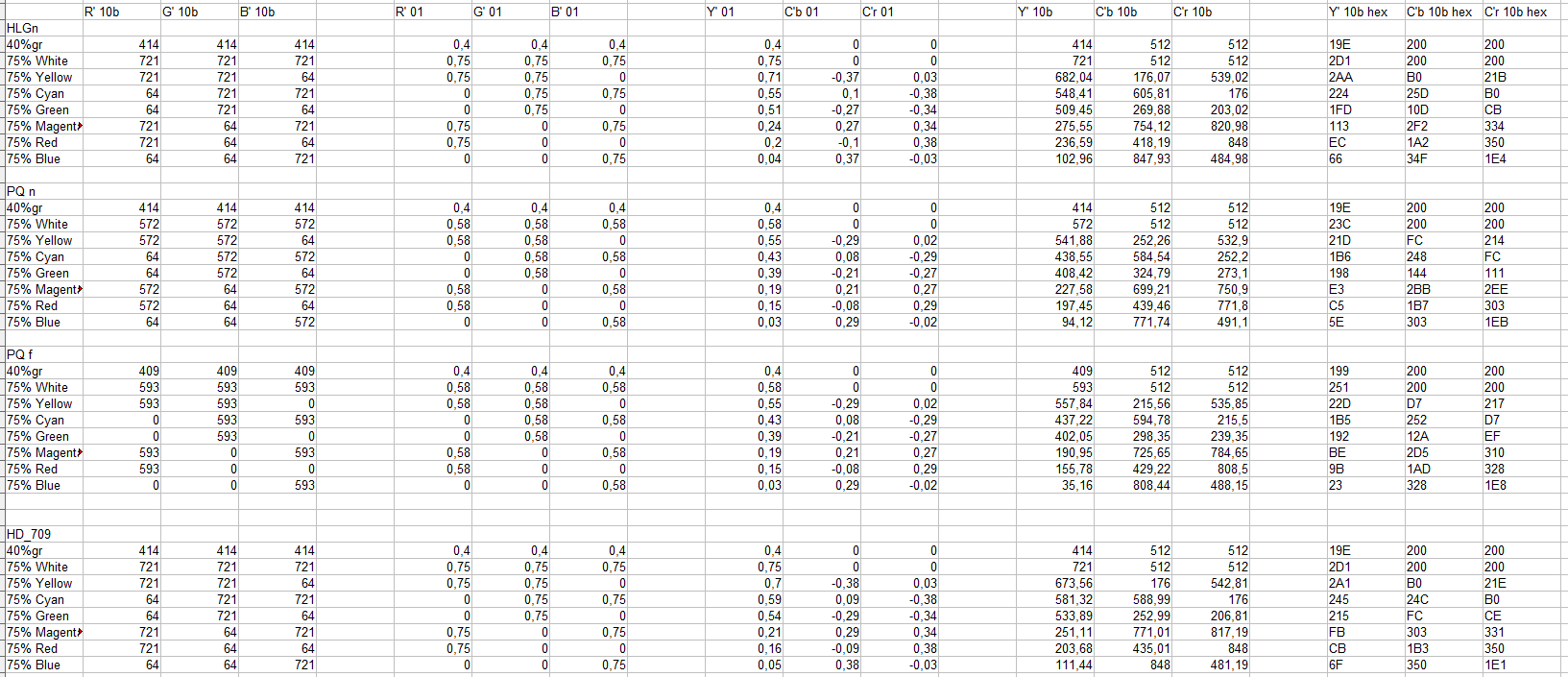"It looks worse to me."

Yes - it have some ringing on horizontal transients. And it is strange because the data after GaussResize have equal conditioning of vertical and horizontal transients.
May be some codevalues distorition happens when transfer from avisynth to vapoursynth. Anyway it may be task to vapoursynth shader designers to check.
Also difference between avs and vps in subtract operation is also with transients shape and levels (causing coloring also)
Code:
```vps=FFMpegSource2("test3shader.mkv")
vps=vps.ConvertToRGB24(matrix="PC.709")
ConvertToRGB24(matrix="PC.709")
Subtract(last,vps)```
Image: https://i5.imageban.ru/out/2021/09/1...054da523c4.png
So it looks there are really lots of codevalues changes somwhere.

Last edited by DTL; 18th September 2021 at 08:23.18th September 2021, 19:53 #23  |  Link DTL Registered User   Join Date: Jul 2018 Posts: 551 It looks I understand why it is so hard to get nice color-bars in subsampled form. The typically good looking only 1 of 3 RGB transients like white-yellow (B-only transient) and cyan-green (B-only transient). But with 2 or 3 transients at once it much more awful. It looks like different rise and fall speed of full-band Y/G and half band R and B makes bright and dark shadows on many color transients. The green-magenta is something like (not very exact/symmethrical created in MS-paint with lines and curves)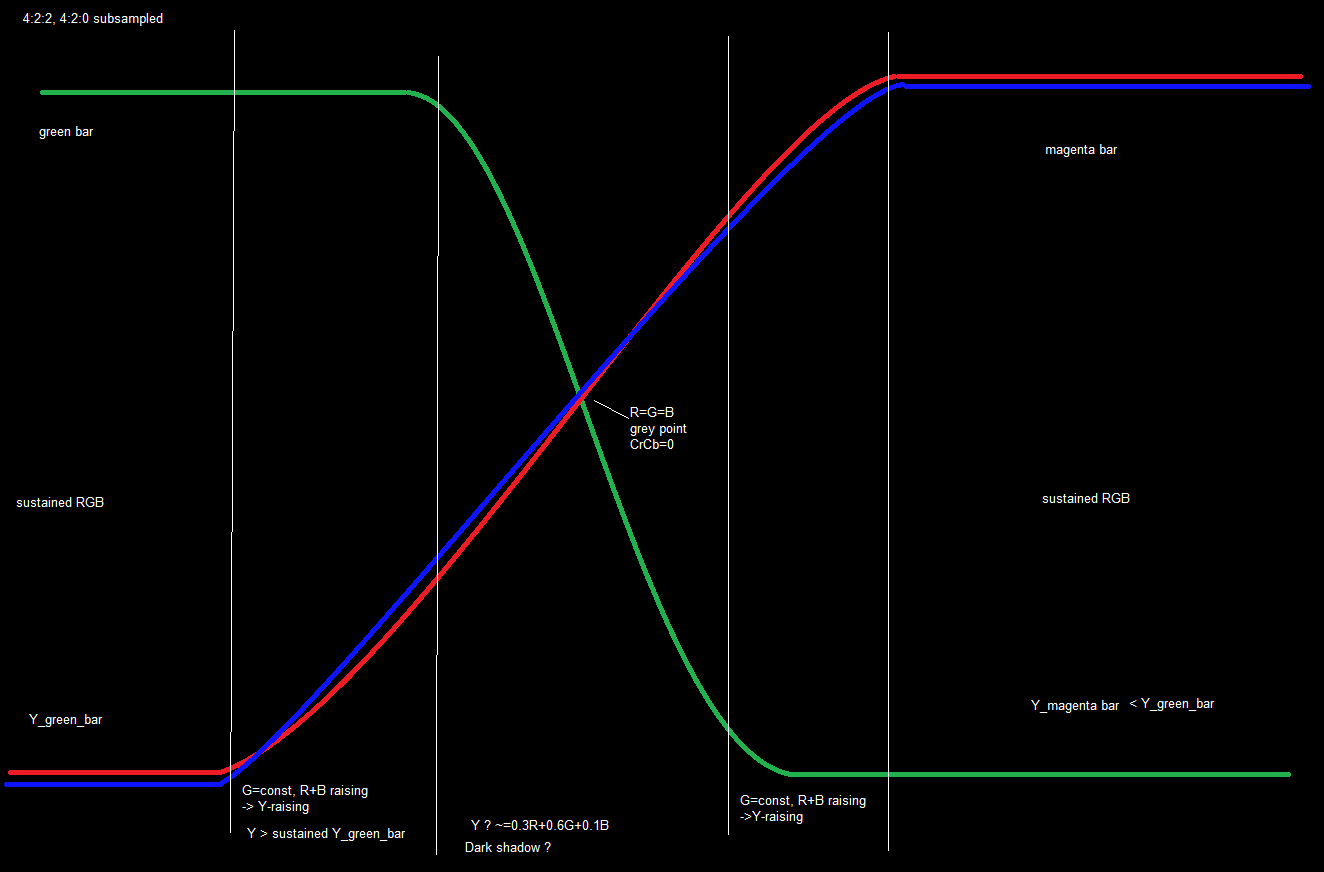So if any component is sub-sample misaligned - we got significant Y-error out of expected shape. So I think of attempt of limiting band of Y so sort of 2:2:2 (in faked 4:2:2) may be look better - need to test. Current result still no good. Last edited by DTL; 18th September 2021 at 19:58.30th September 2021, 00:23 #24  |  Link DTL Registered User   Join Date: Jul 2018 Posts: 551 Made 2 tools for manual transients design (in SDR and PQ/HLG HDR transfers/2020-matrix): Web-based simulator https://github.com/DTL2020/TransientsDesign Tool for write raw files from text-defined samples: https://github.com/DTL2020/WriteTransients It have built-in chroma anti-ring filter I hope close to UserDefined2Resize(b=105,c=0). Green-magenta transient in SDR looks like https://i1.imageban.ru/out/2021/09/3...591a84e339.png So typical display-simulator script is: Code: ```Function Convert422ToRGB24mon_lin_x4_709(clip c) { uc=UToY(c) vc=VToY(c) uc=UserDefined2ResizeMT(uc,src_left=0.001,uc.width, uc.height, b=105, c=0) vc=UserDefined2ResizeMT(vc,src_left=0.001,vc.width, vc.height, b=105, c=0) uc=SincLin2ResizeMT(uc, src_left=0, uc.width*2, uc.height, taps=8) vc=SincLin2ResizeMT(vc, src_left=0, vc.width*2, vc.height, taps=8) yuv444=CombinePlanes(c, uc, vc, planes="YUV", source_planes="YYY", pixel_type="YUV444P10") rgb_lin=ConvertYUVToLinearRGB(yuv444,Color=2) rgb_lin=SincLin2ResizeMT(rgb_lin,rgb_lin.width*4, rgb_lin.height*4, taps=16) yuv_out=ConvertLinearRGBToYUV(rgb_lin,Color=2) return yuv_out.ConvertToRGB24(matrix="PC.709") }``` Chroma position relative to Y' looks like not require 0.5..1 sample shift at conversion to 4:4:4 (not sure if it correct).2nd October 2021, 19:56 #25  |  Link DTL Registered User   Join Date: Jul 2018 Posts: 551 Now with ability to put transient sourcing to scripting (instead of loading external raw-file) it is easier to test different HLG to linear convertors (for monitoring). Code: ```LoadPlugin("plugins_JPSDR.dll") LoadPlugin("avsresize.dll") LoadPlugin("fmtcavs.dll") Function Convert422ToRGB24mon_lin_x4_HLG(clip c) { uc=UToY(c) vc=VToY(c) uc=UserDefined2ResizeMT(uc,src_left=0.001,uc.width, uc.height, b=105, c=0) vc=UserDefined2ResizeMT(vc,src_left=0.001,vc.width, vc.height, b=105, c=0) uc=SincLin2ResizeMT(uc, src_left=0, uc.width*2, uc.height, taps=8) vc=SincLin2ResizeMT(vc, src_left=0, vc.width*2, vc.height, taps=8) yuv444=CombinePlanes(c, uc, vc, planes="YUV", source_planes="YYY", pixel_type="YUV444P10") # jpsdr_plugin # rgb_lin=ConvertYUVtoLinearRGB(yuv444,Color=0,HDRMode=2) # rgb_lin=RGBAdjust(rgb_lin,r=3,g=3,b=3) # avsresize # rgb_lin=z_convertformat(yuv444,pixel_type="rgbps", colorspace_op="2020ncl:std-b67:2020:l=>rgb:linear:2020:l", nominal_luminance=300) #fmtconv rgb_lin=fmtc_matrix(yuv444,mat="RGB", mats="2020",fulls=true, fulld=true) rgb_lin=fmtc_transfer(rgb_lin,transs="hlg",transd="linear",bits=32) rgb_lin=RGBAdjust(rgb_lin,r=3,g=3,b=3) rgb_lin=SincLin2ResizeMT(rgb_lin,rgb_lin.width*4, rgb_lin.height*4, taps=16) yuv_out=ConvertLinearRGBToYUV(rgb_lin,Color=2) return yuv_out.ConvertToRGB24(matrix="PC.709") } plY=Blankclip(width = 10, height = 1, pixel_type="Y10").Expr("sx 0 == 509 sx 1 == 509 sx 2 == 509 sx 3 == 509 sx 4 == 509 sx 5 = 498 sx 6 = 446 sx 7 == 361 sx 8 == 292 276 ? ? ? ? ? ? ? ? ?") plU=Blankclip(width = 5, height = 1, pixel_type="Y10").Expr("sx 0 == 270 sx 1 == 263 sx 2 == 330 sx 3 == 761 754 ? ? ? ?") plV=Blankclip(width = 5, height = 1, pixel_type="Y10").Expr("sx 0 == 203 sx 1 == 224 sx 2 == 271 sx 3 == 832 821 ? ? ? ?") tr=CombinePlanes(plY, plU, plV, "YUV", "YYY", pixel_type="YUV422P10") sust_l=Blankclip(width = 4, height = 1, pixel_type="YUV422P10").Expr("sx 0 == 509 sx 1 == 509 sx 2 == 509 509 ? ? ?","sx 0 == 270 270 ?","sx 0 == 203 203 ?").PointResize(50,1) sust_r=Blankclip(width = 4, height = 1, pixel_type="YUV422P10").Expr("sx 0 == 276 sx 1 == 276 sx 2 == 276 276 ? ? ?","sx 0 == 754 754 ?","sx 0 == 821 821 ?").PointResize(52,1) StackHorizontal(sust_l,tr) StackHorizontal(last,sust_r) PointResize(width, 100) # source finishes here, 75% green-magenta transient HLG 10bit 4:2:2 #test convert to 4:4:4 and back #ConvertToYUV444() #ConvertToYUV422() Convert422ToRGB24mon_lin_x4_HLG()``` Results of decoding same green-magenta 75% transient to linear RGBPS by different tools are a bit different: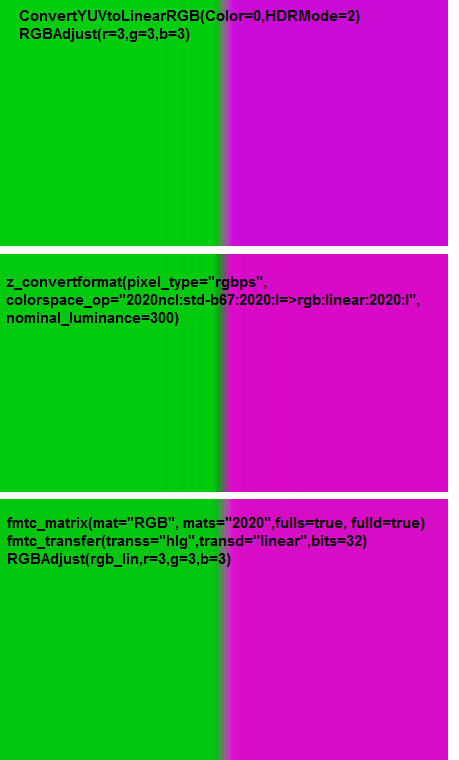jpsdr plugin and fmtc looks close enough and z_convertformat looks like differs most. May be its 'std-b67' is not really linear but also contain OOTF ? Currently the most close to as expected result is from fmtc. Both jpsdr convert and fmtc require RGB gain 3.0 to bring intensity to something >50%. z_convertformat have some misterious param 'nominal_luminance' that greatly infuence the output result but no adjustment for me can bring result close to the other 2 tools. Last edited by DTL; 2nd October 2021 at 20:02. Reason: typo correct3rd October 2021, 06:41   #26  |  Link
StvG
Registered User

Join Date: Jul 2018
Posts: 301
Quote:
 Originally Posted by DTLjpsdr plugin and fmtc looks close enough and z_convertformat looks like differs most. May be its 'std-b67' is not really linear but also contain OOTF ?
https://github.com/sekrit-twc/zimg/i...ment-319853981

approximate_gamma also changes the output.3rd October 2021, 11:18 #27  |  Link DTL Registered User   Join Date: Jul 2018 Posts: 551 "approximate_gamma also changes the output." Yes - it significally changes sustained levels and transient. Looks make things better.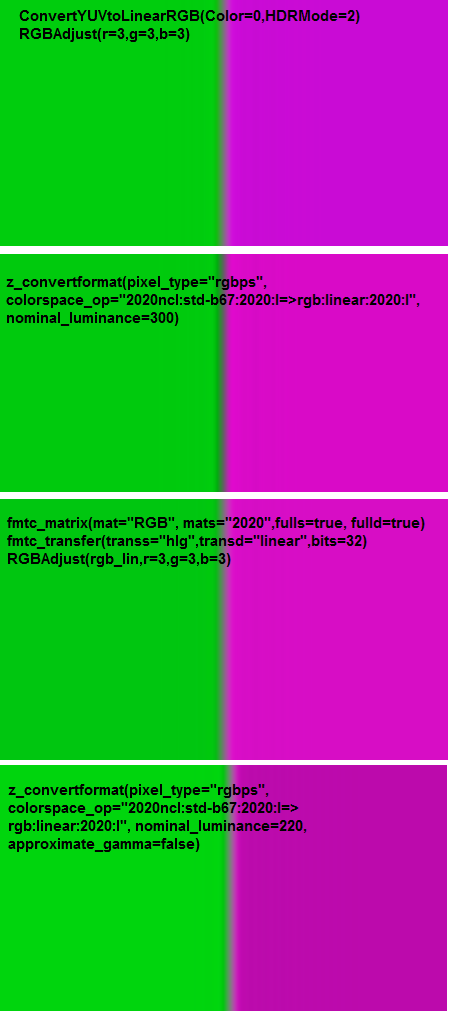Strange why default is not 'false'. Desciption at http://avisynth.nl/index.php/Avsresize says only about reduced precision. I think it is something about 1 LSB error in 16bit. But it may be slower so default is 'true' for speed ? Anyway - one HLG-encoded dataset, one processing path and so different results with different conversion HLG to linear tools. It looks HDR workflows is still a big mess. The colour tone and saturation of magenta field also different a lot. May be de-matrix transforms also not very equal ? I tried to set available control params for HLG to linear conversion to get both good looking of transient and sustained levels of green and magenta. May be better to get best possible RGB levels of sustained green (R=B=0 G=some_level) and magenta (R=B=same_some_level G=0) and look how transient rendering will be. Last edited by DTL; 3rd October 2021 at 11:40.3rd October 2021, 16:22 #28  |  Link StvG Registered User   Join Date: Jul 2018 Posts: 301 Yes, approximate_gamma=false is slow. That's the reason to be true by default. I suggest to use the filter for yuv_HLG->linear to reverse linear->yuv too. So for avsresize: Code: ```... # avsresize # rgb_lin=z_convertformat(yuv444,pixel_type="rgbps", colorspace_op="2020ncl:std-b67:2020:l=>rgb:linear:2020:l", nominal_luminance=1000, approximate_gamma=false) ... yuv_out=z_ConvertFormat(rgb_lin, pixel_type="yuv444ps", colorspace_op="rgb:linear:2020:l=>709:709:709:l") ...``` Now you have different output again. Something about mixing of filters: I would suggest to not use avsresize/ftmconv (didn't tested the last) to perfrom PQ->linear and then tonemapping with HDRTools. If HDRTools is used for tonemapping, use this filter for everything (yuv->linear->tonemapping). If DGx filters are used for tonemapping, use avsresize for yuv->linear. Last edited by StvG; 3rd October 2021 at 16:55. Reason: Was thinkng of PQ while writing about HLG3rd October 2021, 17:15 #29  |  Link DTL Registered User   Join Date: Jul 2018 Posts: 551 "Now you have different output again." Yes - in this script we not control result in linear RGB but pack it again to rec.709 system to feed close to rec.709 typical display (at 2021 year). So may be additional errors. "yuv_out=z_ConvertFormat(rgb_lin, pixel_type="yuv444ps", colorspace_op="rgb:linear:2020:l=>709:709:709:l")" It looks again some misunderstanding. We test colorbars defined as 0..HLG_inverse(0.75) RGB triplets (and transients between this sustained values). Its numbers may define any physical primaries. But when we command system to convert prmaries from 2020 to 709 it creates mess of RGB triplets (also may be also some limited by 709 top values because 2020 colour space > 709 colour space and 0.75 100% saturated HLG is most possibly out of 709 colour space). So all transients will be damaged too. We are not monitor exact rec.2020 physical spectrum (it is not possible on 709 monitors) but want to check if transients do not have ringing/large over/under shooting, parasitic bright or dark lines etc. So to try it we 'cast' rec.2020 RGB triplets to our physical rec.709 display output. It will display rec.709 spectrum colourbars but I hope the Intensity on transients will more or less follow the output of real rec.2020 primaries display. Though in best case it need to be checked with colorimetry calculation. So for 'casting' decoded RGB linear primaries to current display's primaries we just do not touch it: Both yuv_out=z_ConvertFormat(rgb_lin, pixel_type="yuv444ps", colorspace_op="rgb:linear:2020:l=>709:709:2020:l") yuv_out=z_ConvertFormat(rgb_lin, pixel_type="yuv444ps", colorspace_op="rgb:linear:709:l=>709:709:709:l") gives the same result. Though it also differs from jpsdr's plugin. Because jpsdr's plugin can not (I do not know) apply only transfer to RGB without matrix convert to YUV it looks no way to test transfer only. We not need to monitor to go down to YUV matriced data and back so better return from monitor function is return z_ConvertFormat(rgb_lin, pixel_type="rgbp8", colorspace_op="rgb:linear:709:l=>rgb:709:709:l").ConvertToRGB() (I do not see how to output interleaved RGB from z_convertformat directly) Or with fmtc: return fmtc_transfer(rgb_lin,transs="linear",transd="709",fulls=true, fulld=true).ConvertBits(8).ConvertToRGB()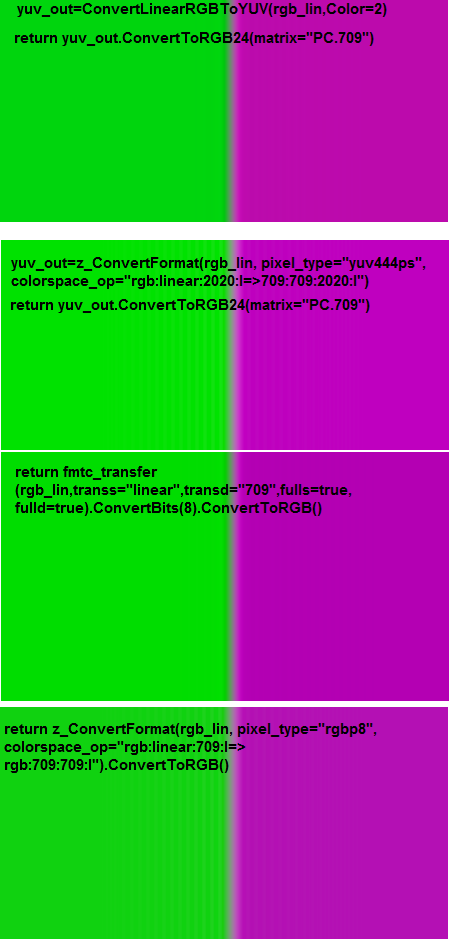It is strange but fmtc again looks the best (almost same as jpsdr's with going to YUV and back). For unknown reason z_convertformat output have lot of low-amplitude ringing (may be it come from x4 upsize or not). Last edited by DTL; 3rd October 2021 at 18:20. Reason: replaced image with more examples3rd October 2021, 20:48 #30  |  Link DTL Registered User   Join Date: Jul 2018 Posts: 551 "I suggest to use the filter for yuv_HLG->linear to reverse linear->yuv too." Why ? When we monitor we do not need to subsample and any more resample - so no additional filtering. The task of processing after getting linear RGB in restored 4x upsampled size is just feed our RGB monitor (that can not still accept linear RGB so we still need to convert RGB to R'G'B' in the transfer of the used monitor that on PC's typically srgb/rec.709). Also at the last simplification if not use jpsdr's plugin it is no more need YUV stage after linear RGB at all.3rd October 2021, 23:35   #31  |  Link
StvG
Registered User

Join Date: Jul 2018
Posts: 301
Quote:
 Originally Posted by DTLBecause jpsdr's plugin can not (I do not know) apply only transfer to RGB without matrix convert to YUV it looks no way to test transfer only.
Right, HDRTools cannot convert only transfer.

Quote:
 Originally Posted by DTL"I suggest to use the filter for yuv_HLG->linear to reverse linear->yuv too." Why ?
I meant the same filter should be used for both operations: convert_to_linear and convert_from_linear.

You're using full range for fmtc bul limited for avsresize.
You can try:
Code:
```# avsresize
rgb_lin=z_convertformat(yuv444,pixel_type="rgbps", colorspace_op="2020ncl:std-b67:2020:f=>rgb:linear:2020:f", nominal_luminance=800)
...
return z_ConvertFormat(rgb_lin, pixel_type="rgbp8", colorspace_op="rgb:linear:709:f=>rgb:709:709:f").ConvertToRGB()```
Code:
```#fmtconv
rgb_lin=fmtc_matrix(yuv444,mat="RGB", mats="2020",fulls=true, fulld=true)
rgb_lin=fmtc_transfer(rgb_lin,transs="hlg",transd="linear",bits=32)
...
return fmtc_transfer(rgb_lin,transs="linear",transd="709",fulls=true, fulld=true).ConvertBits(8).ConvertToRGB()```4th October 2021, 10:49 #32  |  Link DTL Registered User   Join Date: Jul 2018 Posts: 551 "I meant the same filter should be used for both operations: " Oh - I think of 'filter' as engineering device/operation for spectrum changing (filtering) and the only applied spatial frequency filter here is for anti-ringing of subsampled UV. And you use Avisynth filter term as any command operation in Avisynth. Well - the Code: ```rgb_lin=z_convertformat(yuv444,pixel_type="rgbps", colorspace_op="2020ncl:std-b67:2020:l=>rgb:linear:2020:l", nominal_luminance=800) rgb_lin=RGBAdjust(rgb_lin,r=3,g=3,b=3) rgb_lin=SincLin2ResizeMT(rgb_lin,rgb_lin.width*4, rgb_lin.height*4, taps=16) return z_ConvertFormat(rgb_lin, pixel_type="rgbp8", colorspace_op="rgb:linear:709:l=>rgb:709:709:l").ConvertToRGB()``` The only 'magic' combination that produce close result to fmtc and jpsdr's plugin (without conversion params adjustments but with RGB gain = 3.0). I later think nominal_luminance may be same adjustment as RGB gain after z_convertformat, but it looks no. So the main important and hidden param to z_convertformat for HLG to linear is "nominal_luminance=800". Last edited by DTL; 4th October 2021 at 10:52.4th October 2021, 17:10 #33  |  Link StvG Registered User   Join Date: Jul 2018 Posts: 301 Why are you using avsresize with limited range but fmtc with full?4th October 2021, 18:15   #34  |  Link
DTL
Registered User

Join Date: Jul 2018
Posts: 551
Quote:
 Originally Posted by StvGWhy are you using avsresize with limited range but fmtc with full?
Oh - it looks my error of too bad reading documentation. I think fulls/fulld = true as of 'limited'.
Also somwhere set it, somwhere forgot. jpsdr's plugin uses default=limited, fmtc_transfer uses default=full(true), fmtc_matrix (depends). z_convertformat have no default ?

With sustained R'G'B' output still a big mess:
I hope the encoded HLG in 'limited' range so output low-RGB (limited 8bit) should be 16 (and max_RGB not exactly defined because of some 3.0 multiplier to RGB linear to make image bright enough, though maxR=maxG=maxB).
Code:
```  rgb_lin=ConvertYUVtoLinearRGB(yuv444,Color=0,HDRMode=2)
.. scale x4
return ConvertLinearRGBToYUV(rgb_lin,Color=2).ConvertToRGB24(matrix="PC.709")```
returns green R'G'B' 0, 205,13, magenta R'G'B' 201,11,213 - I think it some matrix and transfer errors ?

Code:
```rgb_lin=z_convertformat(yuv444,pixel_type="rgbps", colorspace_op="2020ncl:std-b67:2020:l=>rgb:linear:2020:l", nominal_luminance=800)

.. scale x4

return z_ConvertFormat(rgb_lin, pixel_type="rgbp8", colorspace_op="rgb:linear:709:l=>rgb:709:709:l").ConvertToRGB()```
returns green R'G'B' 16,211,16 and magenta R'G'B' 212,16,212 that looks most perfect and as expected.

Code:
```  rgb_lin=fmtc_matrix(yuv444,mat="RGB", mats="2020",fulls=false, fulld=false)
rgb_lin=fmtc_transfer(rgb_lin,transs="hlg",transd="linear",bits=32,fulls=false, fulld=false)

.. scale x4

return fmtc_transfer(rgb_lin,transs="linear",transd="709",fulls=false, fulld=false).ConvertBits(8).ConvertToRGB()```
returns green R'G'B' 0,227,0 and magenta R'G'B' 228,0,228 that looks like somewhere scaled to 'full' though I everywhere set full*=false. May I need to report issue to fmtc development thread ? (post a question - https://forum.doom9.org/showthread.p...94#post1953894 ).

Last edited by DTL; 4th October 2021 at 18:43.4th October 2021, 20:39 #35  |  Link DTL Registered User   Join Date: Jul 2018 Posts: 551 Oh - the fmtc require some (undocumented magic) of Avisynth to work as expected: Code: `return fmtc_transfer(rgb_lin,transs="linear",transd="709",fulls=false, fulld=false).ConvertBits(8,fulls=true,fulld=false).ConvertToRGB()` Now it outputs also green R'G'B'=16,211,16 and magenta R'G'B' =211,16,211. Need post request for update docs at http://avisynth.nl/index.php/ConvertBits . Current HLG monitor function now: Code: ```Function Convert422ToRGB24mon_lin_x4_HLG(clip c) { uc=UToY(c) vc=VToY(c) uc=UserDefined2ResizeMT(uc,src_left=0.001,uc.width, uc.height, b=105, c=0) vc=UserDefined2ResizeMT(vc,src_left=0.001,vc.width, vc.height, b=105, c=0) uc=SincLin2ResizeMT(uc, src_left=0, uc.width*2, uc.height, taps=8) vc=SincLin2ResizeMT(vc, src_left=0, vc.width*2, vc.height, taps=8) yuv444=CombinePlanes(c, uc, vc, planes="YUV", source_planes="YYY", pixel_type="YUV444P10") # jpsdr_plugin # rgb_lin=ConvertYUVtoLinearRGB(yuv444,Color=0,HDRMode=2) # rgb_lin=RGBAdjust(rgb_lin,r=3,g=3,b=3) # avsresize #rgb_lin=z_convertformat(yuv444,pixel_type="rgbps", colorspace_op="2020ncl:std-b67:2020:l=>rgb:linear:2020:l", nominal_luminance=800) #rgb_lin=RGBAdjust(rgb_lin,r=3,g=3,b=3) #fmtconv rgb_lin=fmtc_matrix(yuv444,mat="RGB", mats="2020",fulls=false, fulld=false) rgb_lin=fmtc_transfer(rgb_lin,transs="hlg",transd="linear",bits=32,fulls=false, fulld=false) rgb_lin=RGBAdjust(rgb_lin,r=3,g=3,b=3) rgb_lin=SincLin2ResizeMT(rgb_lin,rgb_lin.width*4, rgb_lin.height*4, taps=16) #return z_ConvertFormat(rgb_lin, pixel_type="rgbp8", colorspace_op="rgb:linear:709:l=>rgb:709:709:l").ConvertToRGB() return fmtc_transfer(rgb_lin,transs="linear",transd="709",fulls=false, fulld=false).ConvertBits(8,fulls=true,fulld=false).ConvertToRGB() # return ConvertLinearRGBToYUV(rgb_lin,Color=2).ConvertToRGB24(matrix="PC.709") }``` fmtc again wins in sustained levels precision (very small - 1LSB at 8bit at some RGB channels). z_convertformat (very small) better with less visual intensity falling at the green-magenta transient. jpsdr's plugin is subject to many questions. And 2 of 3 full-3 primaries changing at transient examples: Code: ```# red-cyan plY=Blankclip(width = 10, height = 1, pixel_type="Y10").Expr("sx 0 == 240 sx 1 == 252 sx 2 == 283 sx 3 == 359 sx 4 == 418 sx 5 = 495 sx 6 = 545 sx 7 == 548 sx 8 == 548 548 ? ? ? ? ? ? ? ? ?") plU=Blankclip(width = 5, height = 1, pixel_type="Y10").Expr("sx 0 == 418 sx 1 == 418 sx 2 == 432 sx 3 == 600 606 ? ? ? ?") plV=Blankclip(width = 5, height = 1, pixel_type="Y10").Expr("sx 0 == 848 sx 1 == 844 sx 2 == 799 sx 3 == 184 176 ? ? ? ?") tr=CombinePlanes(plY, plU, plV, "YUV", "YYY", pixel_type="YUV422P10") sust_l=Blankclip(width = 4, height = 1, pixel_type="YUV422P10").Expr("sx 0 == 237 sx 1 == 237 sx 2 == 237 237 ? ? ?","sx 0 == 418 418 ?","sx 0 == 848 848 ?").PointResize(50,1) sust_r=Blankclip(width = 4, height = 1, pixel_type="YUV422P10").Expr("sx 0 == 548 sx 1 == 548 sx 2 == 548 548 ? ? ?","sx 0 == 606 606 ?","sx 0 == 176 176 ?").PointResize(52,1) StackHorizontal(sust_l,tr) StackHorizontal(last,sust_r) rc=PointResize(width, 100) # source finishes here, 75% red-cyan transient HLG 10bit 4:2:2 # blue-yellow plY=Blankclip(width = 10, height = 1, pixel_type="Y10").Expr("sx 0 == 103 sx 1 == 103 sx 2 == 143 sx 3 == 294 sx 4 == 416 sx 5 = 568 sx 6 = 659 sx 7 == 684 sx 8 == 682 682 ? ? ? ? ? ? ? ? ?") plU=Blankclip(width = 5, height = 1, pixel_type="Y10").Expr("sx 0 == 848 sx 1 == 848 sx 2 == 710 sx 3 == 202 169 ? ? ? ?") plV=Blankclip(width = 5, height = 1, pixel_type="Y10").Expr("sx 0 == 485 sx 1 == 485 sx 2 == 507 sx 3 == 539 539 ? ? ? ?") tr=CombinePlanes(plY, plU, plV, "YUV", "YYY", pixel_type="YUV422P10") sust_l=Blankclip(width = 4, height = 1, pixel_type="YUV422P10").Expr("sx 0 == 103 sx 1 == 103 sx 2 == 103 103 ? ? ?","sx 0 == 848 848 ?","sx 0 == 485 485 ?").PointResize(50,1) sust_r=Blankclip(width = 4, height = 1, pixel_type="YUV422P10").Expr("sx 0 == 682 sx 1 == 682 sx 2 == 682 682 ? ? ?","sx 0 == 176 176 ?","sx 0 == 539 539 ?").PointResize(52,1) StackHorizontal(sust_l,tr) StackHorizontal(last,sust_r) by=PointResize(width, 100) # source finishes here, 75% blue-yellow transient HLG 10bit 4:2:2``` Looks not very great on simulator but at the monitoring looks better (additionally manually tweaked to look best at monitoring). They are not exist in 'old standard color bars' but possibly useful for testing too. Last edited by DTL; 4th October 2021 at 21:37.5th October 2021, 01:15 #36  |  Link StvG Registered User   Join Date: Jul 2018 Posts: 301 avsresize has the following default values for range: - rgb - full - yuv < 32-bit - limited - yuv 32-bit - full. Both avsresize and fmtc treat a clip in float in the same way - always full range. To avoid range mess and to perform also matrix conversion with fmtc in float: Code: ```# avsresize # rgb_lin=ConvertBits(yuv444, 32, fulls=false, fulld=true).z_convertformat(pixel_type="rgbps", colorspace_op="2020ncl:std-b67=>rgb:linear", nominal_luminance=800) # rgb_lin=RGBAdjust(rgb_lin,r=3,g=3,b=3) #fmtconv rgb_lin=ConvertBits(yuv444, 32, fulls=false, fulld=true).fmtc_matrix(mat="RGB", mats="2020") rgb_lin=fmtc_transfer(rgb_lin,transs="hlg",transd="linear") rgb_lin=RGBAdjust(rgb_lin,r=3,g=3,b=3) ... # return z_ConvertFormat(rgb_lin, colorspace_op="rgb:linear=>rgb:709").ConvertBits(8, fulls=true, fulld=false).ConvertToRGB() return fmtc_transfer(rgb_lin,transs="linear",transd="709").ConvertBits(8, fulls=true, fulld=false).ConvertToRGB() ...```5th October 2021, 13:00 #37  |  Link DTL Registered User   Join Date: Jul 2018 Posts: 551 "fmtc_matrix(mat="RGB", mats="2020")" Yes - the most of full*=false function arguments to fmtc are either useless or may be even make things worse. Unfortunately Avisynth API/environment looks like have not ability to display user-side useful warning messages about arguments interpreting, formats and other. Only can throw stop-errors messages.28th June 2022, 16:29 #38  |  Link DTL Registered User   Join Date: Jul 2018 Posts: 551 Some interesting update after I finally was not too patient to wait more years untill official release of EBU Tech 3325 Test Pattern 7 and decide to ask jpsdr in the mid of 2022 about why it outputs not perfectly matched result with fmtconv and avsresize: https://forum.doom9.org/showthread.p...95#post1970995 He point to some more magic in HDRtools: With Code: ```rgb_lin=ConvertYUVtoLinearRGB(yuv444,Color=0,HDRMode=2, OOTF=false, HLGLw=350) return ConvertLinearRGBToYUV(rgb_lin,Color=2, OOTF=false).ConvertToRGB24(matrix="PC.709")``` Conversion to linear and scaling and back to rec.709 transfer the sustained levels are close enough to avsresize and fmtconv plugins but the view of transient looks different (and may be close to the initial design of this transient in simulator - less contrast dark stripe):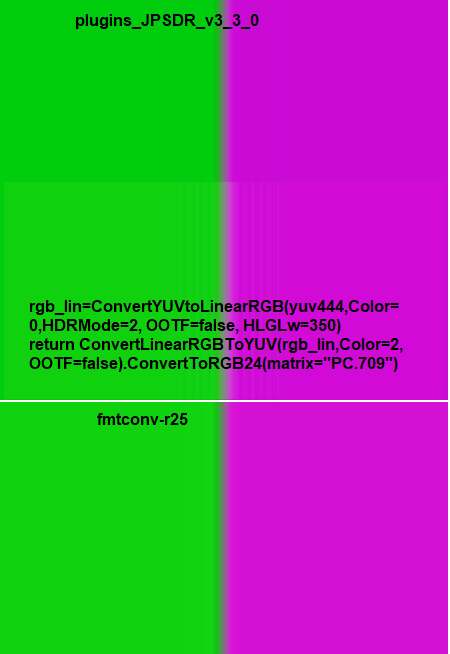Though the Lw=350 is completely adjusted by hand value not based on any theory math just to attempt to put green and magenta sustained levels to match other plugins as close as possible. Compare with old script of 2021 year. So it looks playing around OOTF and Lw values may give different results in the intermediate levels calcultaion. Are there ways in fmtconv and or avsresize to play also with OOTF on/off and Lw adjustment ? Last edited by DTL; 28th June 2022 at 16:36.4th August 2022, 13:49 #39  |  Link StvG Registered User   Join Date: Jul 2018 Posts: 301 The following two conversions give me close output: Code: ```# jpsdr_plugin rgb_lin=ConvertYUVtoLinearRGB(yuv444,Color=0,OOTF=false, HDRMode=2) rgb_lin=RGBAdjust(rgb_lin,r=3,g=3,b=3) rgb_lin=SincLin2ResizeMT(rgb_lin,rgb_lin.width*4, rgb_lin.height*4, taps=16) return ConvertLinearRGBToYUV(rgb_lin,Color=2, OOTF=false).ConvertToRGB24(matrix="PC.709")``` Code: ```# avsresize rgb_lin=z_convertformat(yuv444,pixel_type="rgbps", colorspace_op="2020ncl:std-b67:2020:l=>rgb:linear:2020:l", nominal_luminance=1500) rgb_lin=RGBAdjust(rgb_lin,r=3,g=3,b=3) rgb_lin=SincLin2ResizeMT(rgb_lin,rgb_lin.width*4, rgb_lin.height*4, taps=16) return z_ConvertFormat(rgb_lin, pixel_type="yuv444p16", colorspace_op="rgb:linear:709:l=>709:709:709:l").ConvertToRGB(matrix="PC.709")``` Also the following two conversions: Code: ```# jpsdr_plugin rgb_lin=ConvertYUVtoLinearRGB(yuv444,Color=0,HDRMode=2) rgb_lin=RGBAdjust(rgb_lin,r=3,g=3,b=3) rgb_lin=SincLin2ResizeMT(rgb_lin,rgb_lin.width*4, rgb_lin.height*4, taps=16) return ConvertLinearRGBToYUV(rgb_lin,Color=2).ConvertToRGB24(matrix="PC.709")``` Code: ```# avsresize rgb_lin=z_convertformat(yuv444,pixel_type="rgbps", colorspace_op="2020ncl:std-b67:2020:l=>rgb:linear:2020:l", nominal_luminance=1000) rgb_lin=RGBAdjust(rgb_lin,r=3,g=3,b=3) rgb_lin=SincLin2ResizeMT(rgb_lin,rgb_lin.width*4, rgb_lin.height*4, taps=16) return z_ConvertFormat(rgb_lin, pixel_type="yuv444p16", colorspace_op="rgb:linear:709:l=>709:709:709:l").ConvertToRGB(matrix="PC.709")``` I cannot get close result with fmtconv. It has sceneref and ambient arg but they didn't change anything.5th August 2022, 21:50 #40  |  Link DTL Registered User   Join Date: Jul 2018 Posts: 551 With updated avsresize r15 plugin https://forum.doom9.org/showthread.p...16#post1972716 and scene_referred param it looks finally the winner in precision but still need some very magic numbers of additional correction to finally output 180/16 codevalues high/low R'G'B' 8bit in green-magenta for HLG narrow: Code: ```# jpsdr_plugin # rgb_lin=ConvertYUVtoLinearRGB(yuv444,Color=0,HDRMode=2, OOTF=false, HLGLw=1000) # rgb_lin=RGBAdjust(rgb_lin,r=3,g=3,b=3) # avsresize rgb_lin=z_convertformat(yuv444,pixel_type="rgbps", colorspace_op="2020ncl:std-b67:2020:l=>rgb:linear:2020:l", scene_referred=true) rgb_lin=RGBAdjust(rgb_lin,r=0.157,g=0.157,b=0.157) #fmtconv # rgb_lin=fmtc_matrix(yuv444,mat="RGB", mats="2020",fulls=false, fulld=false) # rgb_lin=fmtc_transfer(rgb_lin,transs="hlg",transd="linear",bits=32,fulls=false, fulld=false) # rgb_lin=RGBAdjust(rgb_lin,r=3,g=3,b=3) rgb_lin=SincLin2ResizeMT(rgb_lin,rgb_lin.width*4, rgb_lin.height*4, taps=16) return z_ConvertFormat(rgb_lin, pixel_type="rgbp8", colorspace_op="rgb:linear:709:l=>rgb:709:709:l").ConvertToRGB() #return fmtc_transfer(rgb_lin,transs="linear",transd="709",fulls=false, fulld=false).ConvertBits(8,fulls=true,fulld=false).ConvertToRGB() #return ConvertLinearRGBToYUV(rgb_lin,Color=2, OOTF=false).ConvertToRGB24(matrix="PC.709")``` also the transient looks about good. jpsdr plugin can only make some unbalanced R'G'B' of Green 16,182,13 and Magenta 171,11,176 when trying to align high value to 180. May be some matrixing is not perfect. Last edited by DTL; 5th August 2022 at 22:09.Thread Tools Search this ThreadShow Printable VersionEmail this Page Search this Thread: Advanced Search Display ModesLinear ModeSwitch to Hybrid ModeSwitch to Threaded ModePosting Rules You may not post new threads You may not post replies You may not post attachments You may not edit your posts BB code is On Smilies are On [IMG] code is On HTML code is Off Forum Rules
 Forum Jump User Control Panel Private Messages Subscriptions Who's Online Search Forums Forums Home Announcements and Chat     General Discussion     News     Forum / Site Suggestions & Help General     Decrypting     Newbies     DVD2AVI / DGIndex     Audio encoding     Subtitles     Linux, Mac OS X, & Co Capturing and Editing Video     Avisynth Usage     Avisynth Development     VapourSynth     Capturing Video     DV     HDTV / DVB / TiVo     NLE - Non Linear Editing     VirtualDub, VDubMod & AviDemux     New and alternative a/v containers Video Encoding     (Auto) Gordian Knot     MPEG-4 ASP     MPEG-4 Encoder GUIs     MPEG-4 AVC / H.264     High Efficiency Video Coding (HEVC)     New and alternative video codecs     MPEG-2 Encoding     VP9 and AV1 (HD) DVD, Blu-ray & (S)VCD     One click suites for DVD backup and DVD creation     DVD & BD Rebuilder     (HD) DVD & Blu-ray authoring     Advanced authoring     IFO/VOB Editors     DVD burning Hardware & Software     Software players     Hardware players     PC Hard & Software Programming and Hacking     Development     Translations

All times are GMT +1. The time now is 01:06.

 Doom9.org - Archive - Top